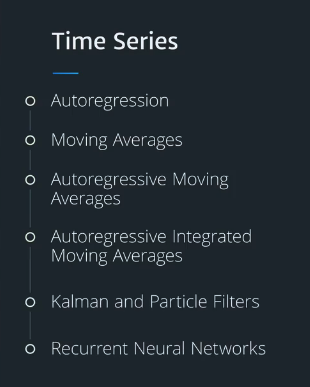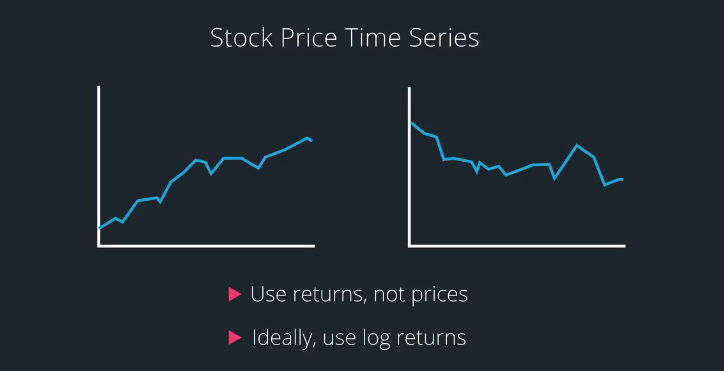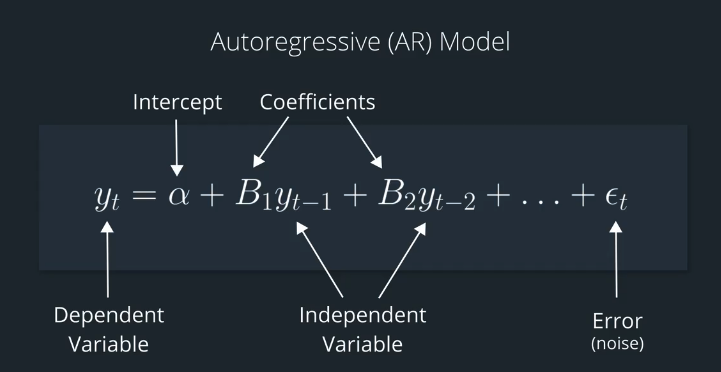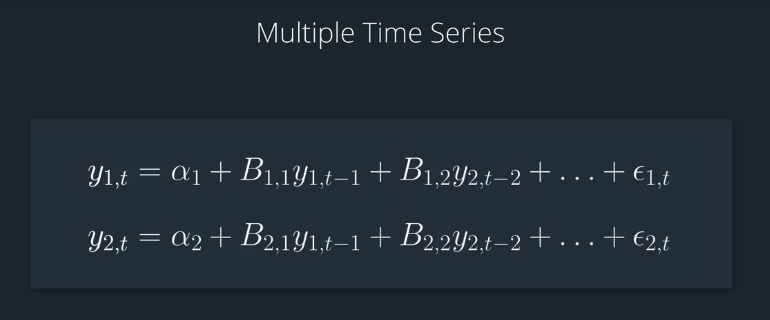# AI For Trading: Time Series Modeling (22)

## Time series analysis

Time series are data that are collected at regular intervals. We will cover two statistical methods, autoregression and moving averages.

This will give us the foundation to cover two more advanced methods,autoregressive moving averages and autoregressive integrated moving averages. From there, we will cover two machine-learning methods.
The first is Kalman filters, and the more generalized, particle filters.
The second is recurrent neural networks.## Autoregressive(AR) Model

We assume that the previous period's value give us some insight into the next period's value.
It's also reasonable to assume that the past couple of data points give us hints as to what the next value will be.

An autoregressive model, also called an AR model, tries to fit a line that is a linear combination of previous values.### Multiple time series## Moving Average Models

Another way to model time series is to think of the stock return hovering around in moving average. As an analogy ,imagine that you're walking at night while holding a lantern, a month files around the lantern,

In the moving average model, often called an MA model